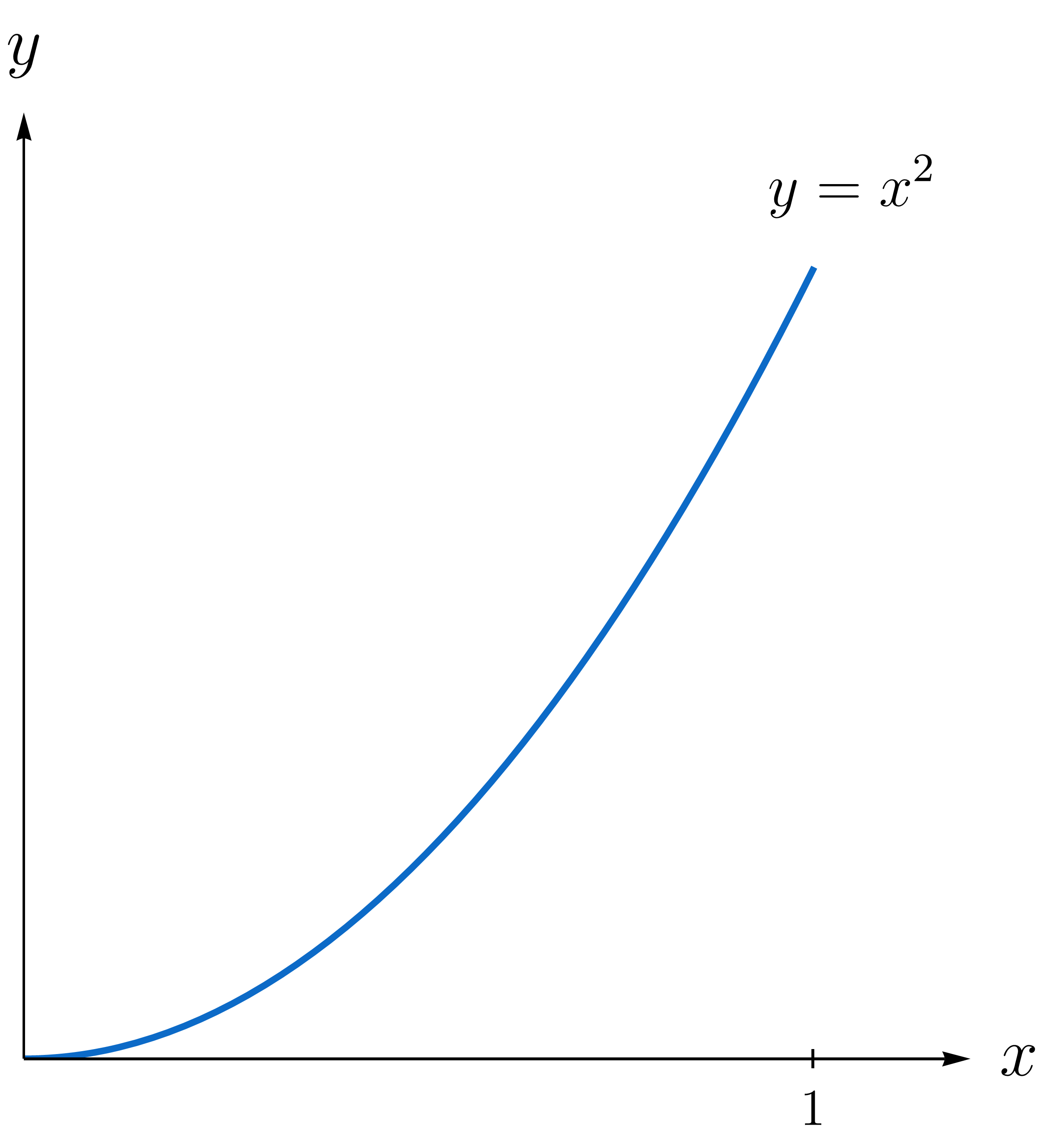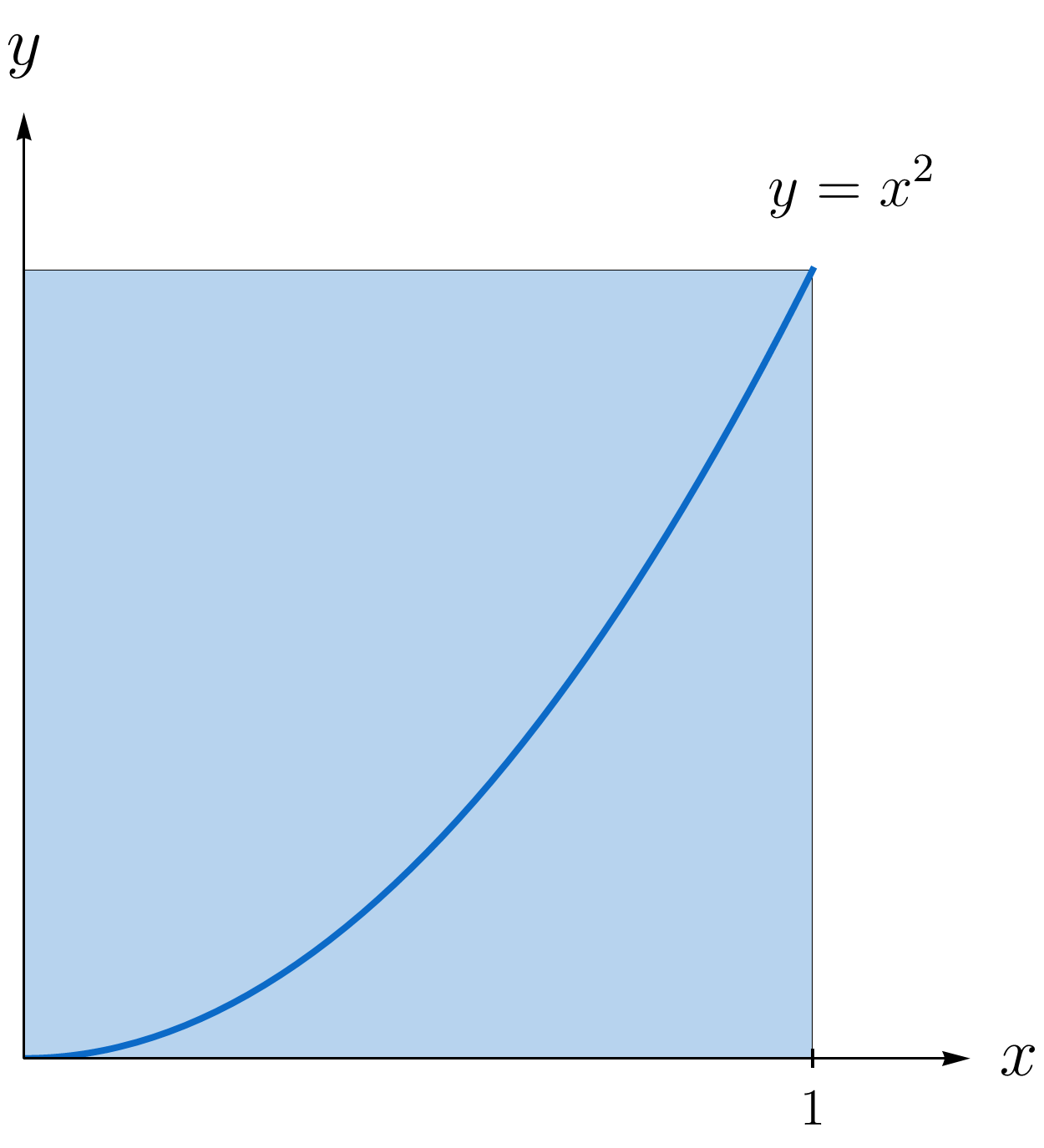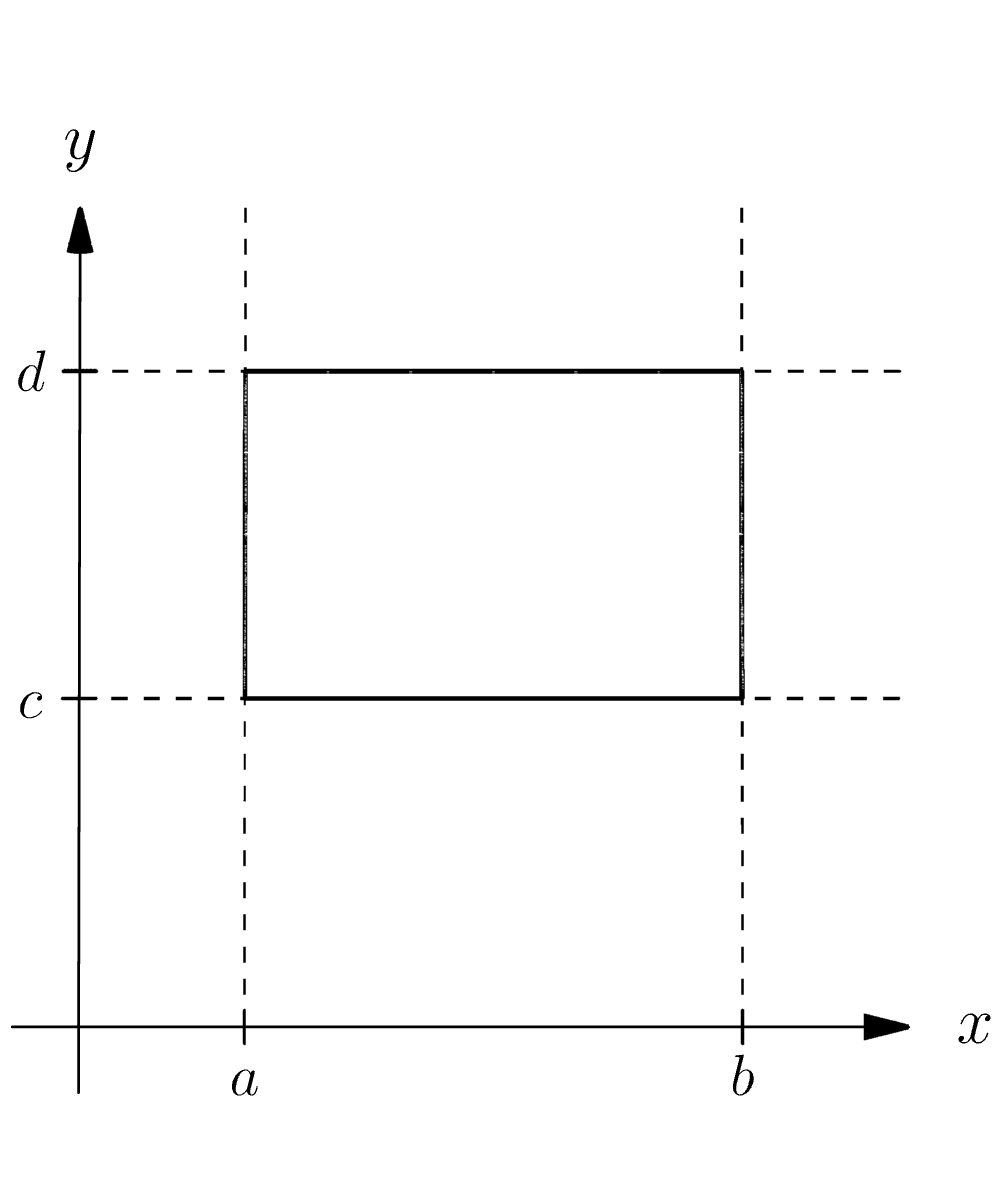### Multivariable Calculus

Single-variable definite integrals are first introduced to compute the area bounded by a set of curves.

The integral has a multitude of applications, but computing a planar area is one of the best visual ways to understand what it means.

It makes sense that the best way to introduce definite integrals of many-variable functions is through their most visual application: computing volumes of 3D regions bounded by surfaces.

We'll use the Riemann sum here to make the jump from single-variable integrals to double integrals, covered in much greater detail in the Multiple Integrals chapter.

# 3D Volumes

A classic problem from single-variable calculus is to compute the area bounded above by the parabola $y = x^2,$ below by the $x$-axis, and between the lines $x = 0$ and $x =1.$We're going to review the process of computing the definite integral $\int\limits_{x=0}^{x=1} x^2 dx .$ The rules of antidifferentiation and the fundamental theorem of calculus gives us the answer in a short calculation. We're not so much interested in the value here as we are in the process.

# 3D Volumes

A definite integral is really the limit of a Riemann sum: $\int\limits_{x=a}^{x=b} f(x)\, dx =\lim\limits_{n \to \infty } \sum\limits_{j=1}^{n} f(x_{j}) \left( \frac{b-a}{n} \right).$ If we break the interval $[a,b]$ up into $n$ equal-sized pieces, $x_{j}$ is the right-hand endpoint of the $j^\text{th}$ subinterval.

What's a general formula for $x_{j}?$

# 3D Volumes

We use thin rectangles of height $f \left(a+ j \left[ \frac{b-a}{n} \right] \right)$ and base length $\frac{b-a}{n}$ to estimate the area of a planar region.

As $n \to \infty$ and more and more rectangles are used, the area approximation gets better and better.The limit (when it exists) is the definite integral $\int\limits_{x=a}^{x=b} f(x) dx =\lim\limits_{n \to \infty } \sum\limits_{j=1}^{n} \underbrace{f \left(a+ j \left[ \frac{b-a}{n} \right] \right)}_{\text{height}} \underbrace{\left( \frac{b-a}{n} \right)}_{\text{base}}.$ Compute $\int\limits_{x=0}^{x=1} x^2 dx$ directly from the Riemann sum using the identity $\sum\limits_{j=1}^{n} j^2 = \frac{n(n+1)(2n+1)}{6}.$

# 3D Volumes

The Riemann sum bridges the gap between single-variable integrals and multiple-variable integrals.

First, let's write down a two variable definite integral; we'll interpret what it means geometrically in a moment: $\int\limits_{x=0}^{x=1} \ \int\limits_{y=0}^{y=1} \left[ x^2+y^2 \right] dx\, dy.$ This looks a lot like the single-variable integral $\int\limits_{x=0}^{x=1} x^2 d x$ we just computed. There, $f(x) = x^2$ measured the height of the point of the curve (parabola) above $x$ on the horizontal axis.

# 3D Volumes

In precisely the same way, $x^2+y^2$ measures the height of a point on a surface directly above $(x,y)$ on the plane.

Below, $p =(a,b,0)$ is an adjustable planar point and $q = (a,b,a^2+b^2)$ is the corresponding point on the surface.

The definite integral $\int\limits_{x=0}^{x=1} \ \int\limits_{y=0}^{y=1} \left[ x^2+y^2 \right] dx\, dy$ is supposed to be the volume contained below this surface and above the square $0 \leq x \leq 1,$ $0 \leq y \leq 1$ in the $xy$-plane.

Note: $0 \leq x ,y \leq 1$ means that both $0 \leq x \leq 1$ and $0 \leq y \leq 1$ are true.

# 3D Volumes

Let's write down a Riemann sum approximating the volume contained below the surface $z = f(x,y) = x^2+y^2$ and above the square $0 \leq x \leq 1,$ $0 \leq y \leq 1.$ First, we'll recap the steps in the single-variable case.

To define$\int_{x=a}^{x=b}f(x)dx,$ we

• break $[a,b]$ into subintervals of length $\frac{b-a}{n}$
• evaluate $f$ at the right-hand endpoints $x_{i} = a + i \frac{b-a}{n}$
• add up the rectangular areas $\text{base} \times \text{height} = \frac{b-a}{n} f(x_{i})$
• then take $n \to \infty.$

Using the animation below as a guide, we can generalize this process:To define $\int_{x=a}^{x=b} \int_{y=c}^{y=d} f(x,y)dydx,$ we

• break the rectangle $a \leq x \leq b, c \leq y \leq d$ into subrectangles of area $\frac{b-a}{n} \frac{d-c}{m}$

• evaluate $f$ at the upper right corners $(x_{i},y_{j} ) = \left( a + i \frac{b-a}{n} , c+j \frac{d-c}{m}\right)$ for $i = 1 , \dots , n$ and $j = 1 , \dots , m$

• add up the volumes $\text{base} \times \text{height} = \frac{b-a}{n} \frac{d-c}{m} f(x_{i},y_{j})$
• then take $m, n \to \infty.$

In short, the Riemann sum approximating the volume has the form $\sum\limits_{i=1}^{n} \sum\limits_{j=1}^{m} \underbrace{f(x_i, y_j)}_{\text{height}} \times \underbrace{\left( \frac{b-a}{n} \frac{d-c}{m} \right)}_{\text{base area}} .$ So what double sum best approximates the volume below the surface $z = f(x,y) = x^2+y^2$ and above the square $0 \leq x \leq 1, 0 \leq y \leq 1\, ?$

# 3D Volumes

The total volume below the surface $z = x^2 +y^2$ and above the square $0 \leq x \leq 1,$ $0 \leq y \leq 1$ is approximately $\sum_{i=1}^{n}\sum_{j=1}^{m} \left ( \frac{i^2}{n^3 m} +\frac{j^2}{m^3 n}\right).$ The exact value is the limit of this approximation as $n , m \to \infty.$

Find the total volume using the identity $\sum\limits_{i=1}^{n} i^2 = \frac{n(n+1)(2n+1)}{6}.$

Note: Before we take $n, m \to \infty,$ the double sums have a finite number of terms. So you can sum over $j$ first while holding $i$ fixed, or vice versa.

For example, \begin{aligned} \sum_{i=1}^{2}\sum_{j=1}^{3} a_{ij} & = \sum_{i=1}^{2} \left( \sum_{j=1}^{3} a_{ij} \right) =\sum_{i=1}^{2} \left( a_{i1}+a_{i2}+a_{i3} \right) \\ & = (a_{11}+a_{12}+a_{13}) + (a_{21}+a_{22}+a_{23}) \\ & = (a_{11}+a_{21})+(a_{12}+a_{22})+(a_{13}+a_{23}) \\ & = \sum_{j=1}^{3} \left( \sum_{i=1}^{2} a_{ij} \right) . \end{aligned}

# 3D Volumes

In single-variable calculus, we learn that the fundamental theorem of calculus can be used to integrate $\int\limits_{x=0}^{x=1} x^2 dx = \int\limits_{x=0}^{x=1} \frac{d}{dx} \left[ \frac{1}{3} x^3 \right] dx = \frac{1}{3} x^3 \Bigg |_{x=0}^{x=1} = \frac{1}{3},$ which matches our Riemann sum result.

Let's see if we can do the same for $\int\limits_{x=0}^{x=1} \ \int\limits_{y=0}^{y=1} \left[ x^2+y^2 \right] dx\, dy.$ This will take some justification later, but assume \begin{aligned} \int\limits_{x=0}^{x=1} \ \int\limits_{y=0}^{y=1} x^2 d x\, dy & = \ \int\limits_{y=0}^{y=1} \left( \int\limits_{x=0}^{x=1} x^2 d x \right) dy \\ \int\limits_{x=0}^{x=1} \ \int\limits_{y=0}^{y=1} y^2 d x\, dy & = \ \int\limits_{x=0}^{x=1} \left( \int\limits_{y=0}^{y=1} y^2 d y \right) dx,\end{aligned} which seems perfectly natural. In words, we integrate in one variable, then the other.

Compute the volume integral using single-variable integration rules.

# 3D Volumes

It seems like the double integral $\int\limits_{x=a}^{x=b} \ \int\limits_{y=c}^{y=d} f(x,y)\, dx\, dy ,$ which represents the volume below the surface $z = f(x,y) \geq 0$ and above the rectangle $a \leq x \leq b , c \leq y \leq d ,$ can be computed using nested single-variable integrals: $\int\limits_{x=a}^{x=b} \left( \int\limits_{y=c}^{y=d} f(x,y)\, dy \right) dx =\int\limits_{y=c}^{y=d} \left( \int\limits_{x=a}^{x=b} f(x,y)\, dx \right) dy.$ Like partial differentiation, when an integral is performed in one variable, the other is held fixed.

If this is valid, find the volume below the surface $z= f(x,y) = x e^{y}$ and above the rectangle $0 \leq x \leq 2 , -1 \leq y \leq 1.$

# 3D Volumes

We used the Riemann sum for a single-variable integral to go one dimension higher into double integration. Such double integrals find the volume of solid regions among many other things.

This is just the beginning of a long story we'll take up again in the Multiple Integrals chapter. There, we'll integrate over more interesting domains, and apply multiple integrals to physics, engineering, and probability problems.

We hope you enjoyed this brief introduction to the basic ideas underlying multivariable calculus.

Our next chapter, Vector Bootcamp, covers the essentials of vectors, one of the basic building blocks of our course.

# 3D Volumes

×